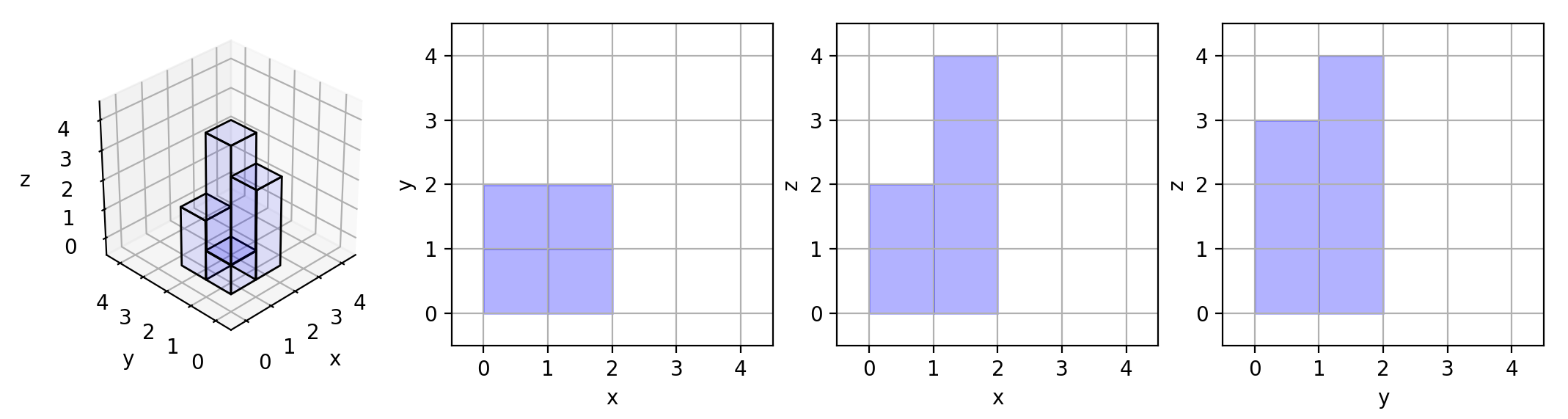### 描述

On a N * N grid, we place some 1 * 1 * 1cubes that are axis-aligned with the x, y, and z axes.

Each value v = grid[i][j] represents a tower of v cubes placed on top of grid cell (i, j).

Now we view the projection of these cubes onto the xy, yz, and zx planes.

A projection is like a shadow, that maps our 3 dimensional figure to a 2 dimensional plane.

Here, we are viewing the “shadow” when looking at the cubes from the top, the front, and the side.

Return the total area of all three projections.

Example 1:

Example 2:Example 3:

Example 4:

Example 5:

Note:

• 1 <= grid.length = grid.length <= 50
• 0 <= grid[i][j] <= 50

### 描述

We are given two sentences A and B. (A sentence is a string of space separated words. Each word consists only of lowercase letters.)

A word is uncommon if it appears exactly once in one of the sentences, and does not appear in the other sentence.

Return a list of all uncommon words.

You may return the list in any order.

Example 1:

Example 2:

Note:

1. 0 <= A.length <= 200
2. 0 <= B.length <= 200
3. A and B both contain only spaces and lowercase letters.

### 描述

Return any binary tree that matches the given preorder and postorder traversals.

Values in the traversals pre and post are distinct positive integers.

Example 1:

Note:

• 1 <= pre.length == post.length <= 30
• pre[] and post[] are both permutations of 1, 2, ..., pre.length.
• It is guaranteed an answer exists. If there exists multiple answers, you can return any of them.

### 描述

The gray code is a binary numeral system where two successive values differ in only one bit.

Given a non-negative integer n representing the total number of bits in the code, print the sequence of gray code. A gray code sequence must begin with 0.

For example, given n = 2, return [0,1,3,2]. Its gray code sequence is:

### 描述

You have a list of words and a pattern, and you want to know which words in words matches the pattern.

A word matches the pattern if there exists a permutation of letters p so that after replacing every letter x in the pattern with p(x), we get the desired word.

(Recall that a permutation of letters is a bijection from letters to letters: every letter maps to another letter, and no two letters map to the same letter.)

Return a list of the words in words that match the given pattern.

You may return the answer in any order.

Example 1:

Note:

• 1 <= words.length <= 50
• 1 <= pattern.length = words[i].length <= 20

### 描述

On a N * N grid, we place some 1 * 1 * 1cubes.

Each value v = grid[i][j] represents a tower of v cubes placed on top of grid cell (i, j).

Return the total surface area of the resulting shapes.

Example 1:

Example 2:

Example 3:

Example 4:

Example 5:

Note:

• 1 <= N <= 50
• 0 <= grid[i][j] <= 50

### 描述

You are given an array A of strings.

Two strings S and T are special-equivalent if after any number of moves, S == T.

A move consists of choosing two indices i and j with i % 2 == j % 2, and swapping S[i] with S[j].

Now, a group of special-equivalent strings from A is a non-empty subset S of A such that any string not in S is not special-equivalent with any string in S.

Return the number of groups of special-equivalent strings from A.

Example 1:

Example 2:

Example 3:

Example 4:

Note:

• 1 <= A.length <= 1000
• 1 <= A[i].length <= 20
• All A[i] have the same length.
• All A[i] consist of only lowercase letters.

### 描述

Given a tree, rearrange the tree in in-order so that the leftmost node in the tree is now the root of the tree, and every node has no left child and only 1 right child.

Note:

1. The number of nodes in the given tree will be between 1 and 100.
2. Each node will have a unique integer value from 0 to 1000.

### 描述

An array is monotonic if it is either monotone increasing or monotone decreasing.

An array A is monotone increasing if for all i <= j, A[i] <= A[j]. An array A is monotone decreasing if for all i <= j, A[i] >= A[j].

Return true if and only if the given array A is monotonic.

### 描述

Given an array A of non-negative integers, return an array consisting of all the even elements of A, followed by all the odd elements of A.

You may return any answer array that satisfies this condition.

Example 1:

Note:

1. 1 <= A.length <= 5000
2. 0 <= A[i] <= 5000